Courses

# Chemistry Test - 9

## 45 Questions MCQ Test | Chemistry Test - 9

Description
This mock test of Chemistry Test - 9 for helps you for every entrance exam. This contains 45 Multiple Choice Questions for Chemistry Test - 9 (mcq) to study with solutions a complete question bank. The solved questions answers in this Chemistry Test - 9 quiz give you a good mix of easy questions and tough questions. students definitely take this Chemistry Test - 9 exercise for a better result in the exam. You can find other Chemistry Test - 9 extra questions, long questions & short questions for on EduRev as well by searching above.
QUESTION: 1

### Which is high spin complex ?

Solution:

Only complex [Cr(gly)3​] is a high spin complex because it contains a weak field ligand and these type of ligands can not pair up the unpaired electrons while in other options there are atleast  one strong field ligand. Hence,option A is correct.

QUESTION: 2

Solution:
QUESTION: 3

### Correct formula of the complex formed in the brown ring test for nitrates is

Solution:
QUESTION: 4

A complex cation is formed by Pt (in same oxidation state) with ligands (in proper number so that coordination number of Pt becomes six). Which of the following can be its correct IUPAC name ?

Solution:
QUESTION: 5

Which of the following is incorrect reaction ?

Solution:
QUESTION: 6

The magnetic moment of a transition metal ion is found to be 3.87 B.M. The number of unpaired electrons present in it is

Solution:
QUESTION: 7

Cl2 gas is dried over

Solution:
QUESTION: 8

Which one of the following pentafluorides cannot be formed ?

Solution:
QUESTION: 9

Which of the following statements is incorrect ?

Solution:
QUESTION: 10

Which one is monobasic acid ?

Solution:
QUESTION: 11

The oxidation state of sulphur in the anions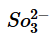,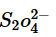,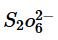follows the order

Solution:
QUESTION: 12

Four metals and their methods of refinement are given
I. Ni, Cu, Zr, Ga
II. electrolysis, van Arkel process, zone refining, Mond’s process
Choose the right method for each

Solution:
QUESTION: 13

Which of the following chemicals is used as a depressant in separating ZnS from PbS in froth floatation process ?

Solution:
QUESTION: 14

Which of the isomeric C4H9Cl compounds will be optically active ?

Solution:
QUESTION: 15

The relative ease of dehydration of following alcohols is
I. CH3CH(OH)CH3
II. (CH3)3COH  III. CH3CH2CH2OH

Solution:
QUESTION: 16

Correct arrangement of the following acids in decreasing order of pKa values is
I. CH3COOH     II. Cl2CHCOOH
III. F3CCOOH   IV. ClCH2COOH
V. FCH2COOH

Solution:
QUESTION: 17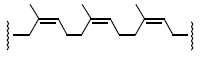The given polymer is

Solution:
QUESTION: 18

Arrange the following
I. CH3CH2CH2Cl
II. CH3CH2CH(Cl)CH3
III. (CH3)2CHCH2Cl
IV. (CH3)3CCl
in order of decreasing tendency towards SN2 reaction

Solution:
QUESTION: 19

Which one of the following reaction is a method for the conversion of a ketone into a hydrocarbon ?

Solution:
QUESTION: 20

The reagent which does not react with both acetone and benzaldehyde is

Solution:
QUESTION: 21

Hinsenberg’s reagent is

Solution:
QUESTION: 22

The van’t Hoff factor for a 0.1 M Al2(SO4)3 solution is 4.20. The degree of dissociation is

Solution:
QUESTION: 23

The product (Z) of the following sequence of reaction is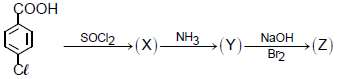Solution:
QUESTION: 24

Match List I with List II and select the correct answer using the codes given below the lists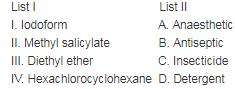Solution:
QUESTION: 25

Such detergent is formed when stearic acid reacts with polytthene glycol.

Solution:
QUESTION: 26

Unit of frequency factor A in k = Ae-Ea/RT  is

Solution:
QUESTION: 27

In which case should N2(g) be more soluble in water?

Solution:
QUESTION: 28

Which of the following does not undergo HVZ reaction ?

Solution:
QUESTION: 29

The two monosaccharides of sucrose are held together by a glycosidic linkage between

Solution:
QUESTION: 30

When ammonia gas is brought in contact with water surface, its pressure falls due to

Solution:
QUESTION: 31

When a solution of AgNO3 (1 M) is electrolysed using platinum anode and copper cathode.What are the products obtained at two electrodes ?
Given :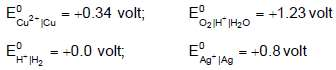Solution:
QUESTION: 32

If the radii of A+ and B- in the crystalline solid AB are 96 pm and 200 pm respectively. The type of voids occupied is

Solution:
QUESTION: 33

Select the incorrect statement among the following,.

Solution:
QUESTION: 34

Which of the following factors is responsible for the decrease in the rate of a surface catalysed reaction?

Solution:
QUESTION: 35

A quantity of iodine was reacted with a large excess of propanone and dilute acid. The equation for the reaction was

I2 + CH3COCH3 → ICH2COCH3 + HI

The concentration of iodine in the reaction mixture was recorded at regular time intervals and the result is shown in figure. Which one the following deductions can be made from this graph ?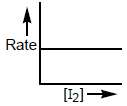Solution:
QUESTION: 36

The equivalent conductance at infinite dilution of a weak acid such as HF

Solution:
QUESTION: 37

A sample of element has 12.08 x 1023 bcc unit cells. Total number of atoms in the given sample are

Solution:
QUESTION: 38

Equivalent conductivity of Fe2(SO4)3 is related to molar conductivity by the expression

Solution:
QUESTION: 39

(R) in the given reaction sequence is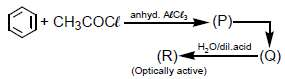Solution:
QUESTION: 40

Which of the following alcohols will yield the corresponding alkyl chloride on reaction with concentrated HCl at room temperature ?

Solution:
QUESTION: 41

Which of the following has ester linkage ?

Solution:
QUESTION: 42

A polymer of prop-2-ene nitrile is called

Solution:
QUESTION: 43

Solution:
QUESTION: 44

Characteristic feature of the side chain of amino acids are given below. Which of the following is the side chain of an essential amino acid ?

Solution:
QUESTION: 45

For the reaction, 2A + B + C → A2B + C, the rate = k[A][B]2 with k = 2.0 x 10-6 mol-2 L2 s-1. Initial concentrations are [A] = 0.1 M, [B] = 0.2 M and [C] = 0.8 M. If the rate of reverse reaction is negligible then, what will be the rate of reaction after [A] is reduced to 0.06 M ?

Solution:

Track your progress, build streaks, highlight & save important lessons and more!

### Similar Content### Related tests